English
Continue in the app
Be The First To Get Support Updates

# FAQ: What is a root tile and how are they used to make a vector tile package with a local coordinate system?

## Question

What is a root tile and how are they used to make a vector tile package with a local coordinate system?

```Note:
Before beginning , consider that the default ArcGIS Online default tiling scheme is the simplest workflow.```

To use a custom scheme, Let’s start by clarifying the requirements of a tiling scheme for use in vector tile creation.

What is the Root Tile?
If a tile cache were a pyramid, the root tile would be its peak. It is the tile that represents the largest area of the map and the lowest level of detail. For example, an ArcGIS Online Basemap that uses the WGS_1984_Web_Mercator_Auxiliary_Sphere coordinate system and the default tiling scheme, the root tile is represented with the “Start Tile” For Level of Detail 0 (LOD 0), as shown on the World Topo Map (MapServer):

• Level ID: 0 [ Start TileEnd Tile ]
• Resolution: 156543.03392800014
• Scale: 5.91657527591555E8

Why are the Scales Doubled?
It is important to note that the Default ArcGIS online tiling scheme has scales that double. Note the second Level of Detail; “Level ID: 1” that the scale value is exactly half of the scale of LOD 0:

• Level ID: 1 [ Start TileEnd Tile ]
• Resolution: 78271.51696399994
• Scale: 2.95828763795777E8

It is equally important to note how the root tile separates in subsequent Levels of Detail, 1 into 4, into 16 tiles, and so forth. This supports a seamless transition in the vector tile layer when zooming, and supports the tile indexing that allows vector tiles to be so efficient.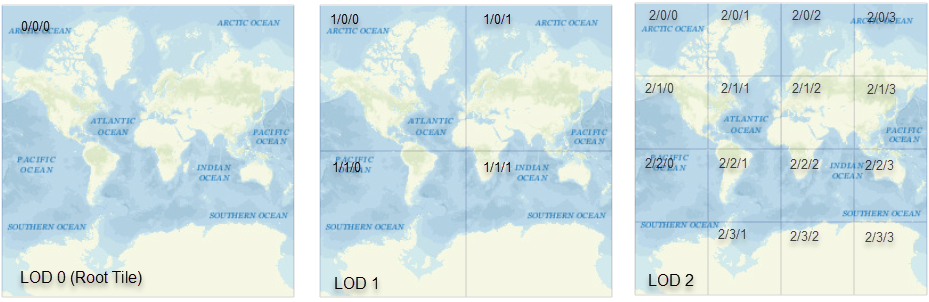How to use the Generate Tile Cache Tiling Scheme tool to make a valid tile scheme for use with vector tiles
See the ArcGIS Pro Help topic: Generate Tile Cache Tiling Scheme

1. Open the Generate Tile Cache Tiling Scheme tool.
2. Set Tile_size to 512 x 512 pixels
3. Set Tile_origin: This identifies the upper left corner of the root tile. This corner should be placed so that the root tile bounding box covers all the data in the map.

In the following image, the map is using the coordinate system “NAD 1983 StatePlane California III FIPS 0403 (US Feet)”, Where the Map Properties show the top left corner as x=5,831,662, y=2,287,211. You can see the placement using the Go to XY tool. This position is used as the Tile_Origin value.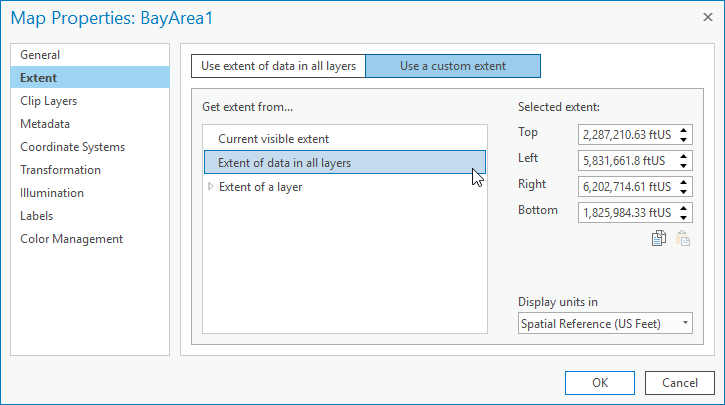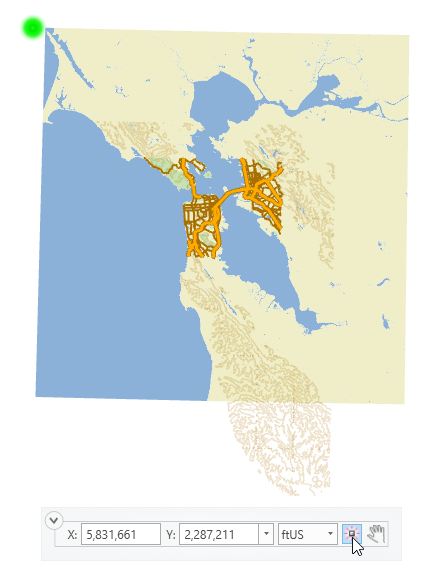1. Set scales: Pick any minimum scale value that suits your data. Each scale must double until a scale is added that surpasses the MaxScale, for example, 1000 > 2000 > 4000 > ...MaxScale > Next Value.
Knowing the bounding box of the root tile is not apparent with the tool parameters, however, use the following equation to reveal MaxScale for the root tile:
```		|Right – Left| = MaxScale*(Tile_size/DPI*Inch2MapUnit)
|Top - Bottom| = MaxScale*(Tile_size/DPI*Inch2MapUnit)

* The bounding box is a square, so go with the MaxScale you find that is larger.
```
Where the extent of the root tile is:
Top= 2287210.63
Left = 5831661.8
Right = 6202714.6
Bottom = 1825984.3
And:
Tile_Size = 512 pixels per tile
DPI = 96 dots (pixels) per inch
Inch2MapUnit = 12 in. to 1 US foot (This may differ depending on the measuring unit of the map's coordinate system)

We subtract the Top and Bottom extents to find the minimum distance the root tile needs to cover is 461,226 feet. So, we use this value to find MaxScale.

Therefore:
```461226.3 = MaxScale*(512/(96*12))
1037758.5 = MaxScale```
Thus, we set the following scales: "2048000;1024000;512000;256000;128000;64000;32000;16000;8000;4000;2000;1000
Note that one additional scale is added because 1037758.5 is slightly bigger then 1024000, therefore we must add an additional scale level for complete coverage.

The following image shows what the final parameters of this example for Generate Tile Cache Tiling Scheme: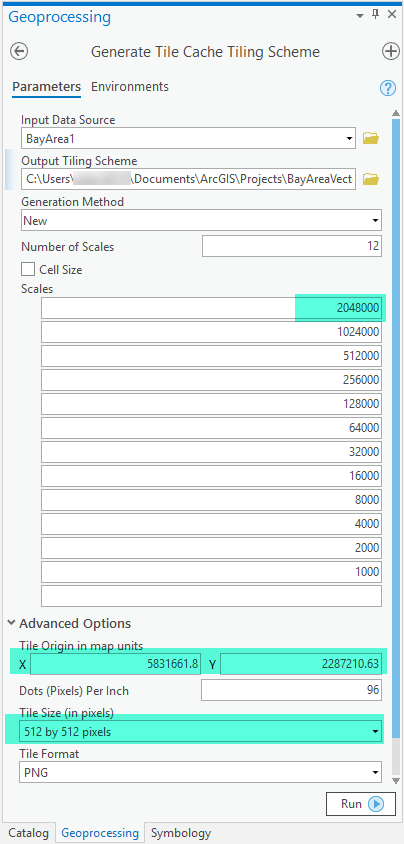The following image is a view of a Vector Tile Index for the example map using this custom tiling scheme: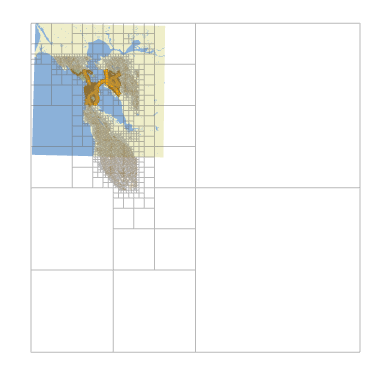## Related Information

Last Published: 9/16/2020

Article ID: 000022396

Software: ArcGIS Pro 2.4.3, 2.4.2, 2.4.1, 2.4